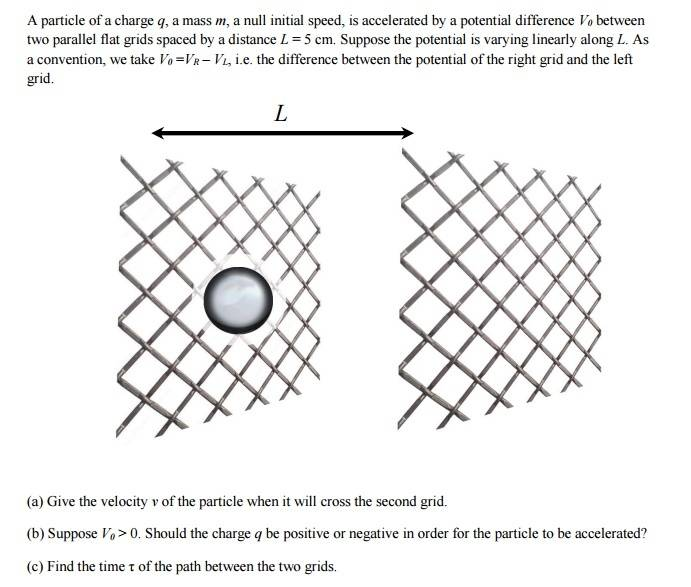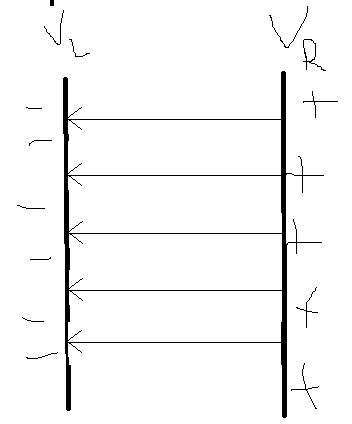# Velocity Charged Particle between Parallel Plates

## Homework StatementCan't really find out b, probably due to a mistake in a. Was wondering what I did wrong.

## Homework Equations

Kinematic equations of motion, w/ constant a
##F=qE##

## The Attempt at a Solution

[/B]
(a)
##E=\frac {\Delta V} {\Delta d}= \frac {V_R-V_L} {L} = \frac {V_0}{L} ##
##F=qE=q \frac {V_0} {L}##
##a=\frac F m =\frac {qV_0} {mL}##
##L=\frac 1 2 a t^2= \frac 1 2 \frac {qV_0} {mL} t^2 \rightarrow t=\sqrt {\frac {2mL^2} {qV_0}}##
##v=at=\frac {qV_0} {mL} \sqrt {\frac {2mL^2} {qV_0}}##

(b)
This is where I'm confused, since filling in a negative charge in this equation will cause solutions for the velocity to be imaginary, which well... Doesn't really work. That makes me guess that the equation is wrong.

(c)
from part (a):
##t=\sqrt {\frac {2mL^2} {qV_0}}##

PS: sidequestion, how could I format those equations better, as they seem too cramped for my liking? I know you can use 's, but that makes the lines spread out too much.

Last edited by a moderator:

gneill
Mentor
The electric field is a vector, and as such has a direction associated with it. When you calculate the force as F = qE there are assumptions being made about how the direction of E relates to your chosen coordinate system.

Make a sketch that includes a vector showing the direction of E when your ##V_o## is greater than zero. What sign should you give E to make it correspond to your choice of coordinate directions?

Well since ##V_0=V_R-V_L## and ##V_0>0## The potential in ##V_R## should be higher than in ##V_L## so we'de get an homogeneous electric field from ##V_R## to ##V_L## like this:Since the charge will go in the direction of the electric field if q is positive, shouldn't we just take a positive sign?

gneill
Mentor
Since the charge will go in the direction of the electric field if q is positive, shouldn't we just take a positive sign?
So if q is positive it will be accelerated to the left, correct? The electric field is pointing to the left. That's a negative acceleration for your coordinate system (which you didn't indicate but I assume the positive x-direction is to the right).

In the above setup a negative charge would be attracted to (accelerate towards) the positive plate.

If you want your "E" to reflect the fact that it points along the negative x-axis direction, you need to give it a negative sign.

Aah, that makes sense. However that leads me to another problem, not being able to find a real value for the time:

##\vec E=\frac {\Delta V} {\Delta d} \hat d=- \frac {V_R-V_L} {L} =- \frac {V_0}{L} ##
##\vec F=q \vec E=-q \frac {V_0} {L}##
##\vec a=\frac {\vec F} {m} =-\frac {qV_0} {mL}##
##L=\frac 1 2 \vec a t^2=- \frac 1 2 \frac {qV_0} {mL} t^2 \rightarrow t^2=-\frac {2mL^2} {qV_0}##

gneill
Mentor
It's not a problem if q is negative. If q is positive the time will have an imaginary solution since a positive charge would never reach the right hand plate.

•ItsTheSebbe
ooh, yeah that does make sense, therefore it needs to negatively charged. I was failing to see the connection between the image and the formula. Thanks!